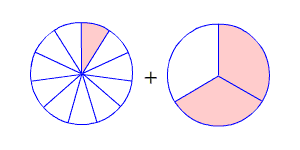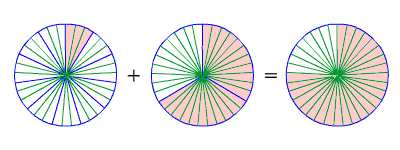# Adding Fractions with Unlike Denominators

If the denominators are not the same, then you have to use equivalent fractions which do have a common denominator . To do this, you need to find the least common multiple (LCM) of the two denominators.

To add fractions with unlike denominators, rename the fractions with a common denominator. Then add and simplify.

For example, suppose you want to add:

$\frac{1}{11}+\frac{2}{3}$The LCM of $3$ and $11$ is $33$ . So, we need to find fractions equivalent to $\frac{1}{11}$ and $\frac{2}{3}$ which have $33$ in the denominator. Multiply the numerator and denominator of $\frac{1}{11}$ by $3$ , and multiply the numerator and denominator of $\frac{2}{3}$ by $11$ .

$\left(\frac{1\text{\hspace{0.17em}}×\text{\hspace{0.17em}}3}{11\text{\hspace{0.17em}}×\text{\hspace{0.17em}}3}\right)+\left(\frac{2\text{\hspace{0.17em}}×\text{\hspace{0.17em}}11}{3\text{\hspace{0.17em}}×\text{\hspace{0.17em}}11}\right)=\frac{3}{33}+\frac{22}{33}$

Now we have like denominators, and we can add as described above.

$=\frac{25}{33}$